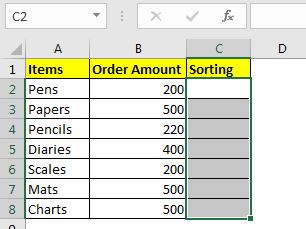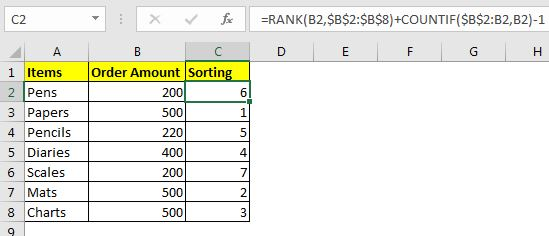# Sort Numeric Values with Excel RANK Function

We have already learned the direct sorting of numeric values in Excel. In this article, we will sort numeric values with the help of Excel RANK function. Here, we will rank each number on the list in a separate column. Then we will sort by that column.

 =RANK(first_number, numbers)+COUNTIF(expanding_range, first_number)-1

numbers: This is the list of numbers that you want to sort. It can be a named range or absolute range.

first_number: This is the relative reference of the first number in numbers. If the numbers are in the range \$A\$1:\$A\$10, then the first_number will be A1

Expanding_range: It is the range that expands relatively when copied. You can read about it here.

Let's see an example of sorting numeric value in excel.

## Example: Rank numbers without duplicates for sorting numbersHere, we have a simple data of some stationary items with their prices. We need to sort this with excel function not by sorting available on the menu. Now to do this we will use the above generic formula.

Write this formula in C2 and drag down (copy to below cells).

 =RANK(B2,\$B\$2:\$B\$8)+COUNTIF(\$B\$2:B2,B2)-1Each item is ranked as per their price. The items that have the same amount are ranked as per their position. There are no ties in the ranking.

How does it work?

This is basically a ranking task, and this can be solely done by the RANK function of excel; if all numbers are unique. But this is not the case here.

To avoid ties and have a unique ranking, we use running count formula (COUNTIF(\$B\$2:B2,B2)) here. We added,

COUNTIF(\$B\$2:B2,B2)-1 to rank formula. This formula will identify if a value occurs again and will add 1 to the rank given by the RANK function to the number.

Now you can use formulas to get sorted results from the list.

So yeah guys, this how you can sort using the Function function. This formula is not intended to give a sorted result. Here we intend to numerically sort in a different column and then use them with other formulas, where this sorting can be a helping hand (helper column). To get sorted results using excel formula use this formula. In Excel 2019, MS has introduced the SORT function which is used to sort values.

Related Articles:

Sort numbers using Excel SMALL function | To sort number ascendingly or descendingly with excel formulas we use the SMALL function along with the ROW. This excel sort formula is accurate and to the point.

Expanding References in Excel | These ranges expand when copied down or to right. They help in running calculations in Excel.

How to do Running Count of Occurance in Excel | Use expanding ranges to count values as per they occur.

Popular Articles:

50 Excel Shortcuts to Increase Your Productivity | Get faster at your task. These 50 shortcuts will make you work even faster on Excel.

The VLOOKUP Function in Excel | This is one of the most used and popular functions of excel that is used to lookup value from different ranges and sheets.

COUNTIF in Excel 2016 | Count values with conditions using this amazing function. You don't need filter your data to count specific value. Countif function is essential to prepare your dashboard.

How to Use the SUMIF Function in Excel | This is another dashboard essential function. This helps you sum up values on specific conditions.

Terms and Conditions of use

The applications/code on this site are distributed as is and without warranties or liability. In no event shall the owner of the copyrights, or the authors of the applications/code be liable for any loss of profit, any problems or any damage resulting from the use or evaluation of the applications/code.